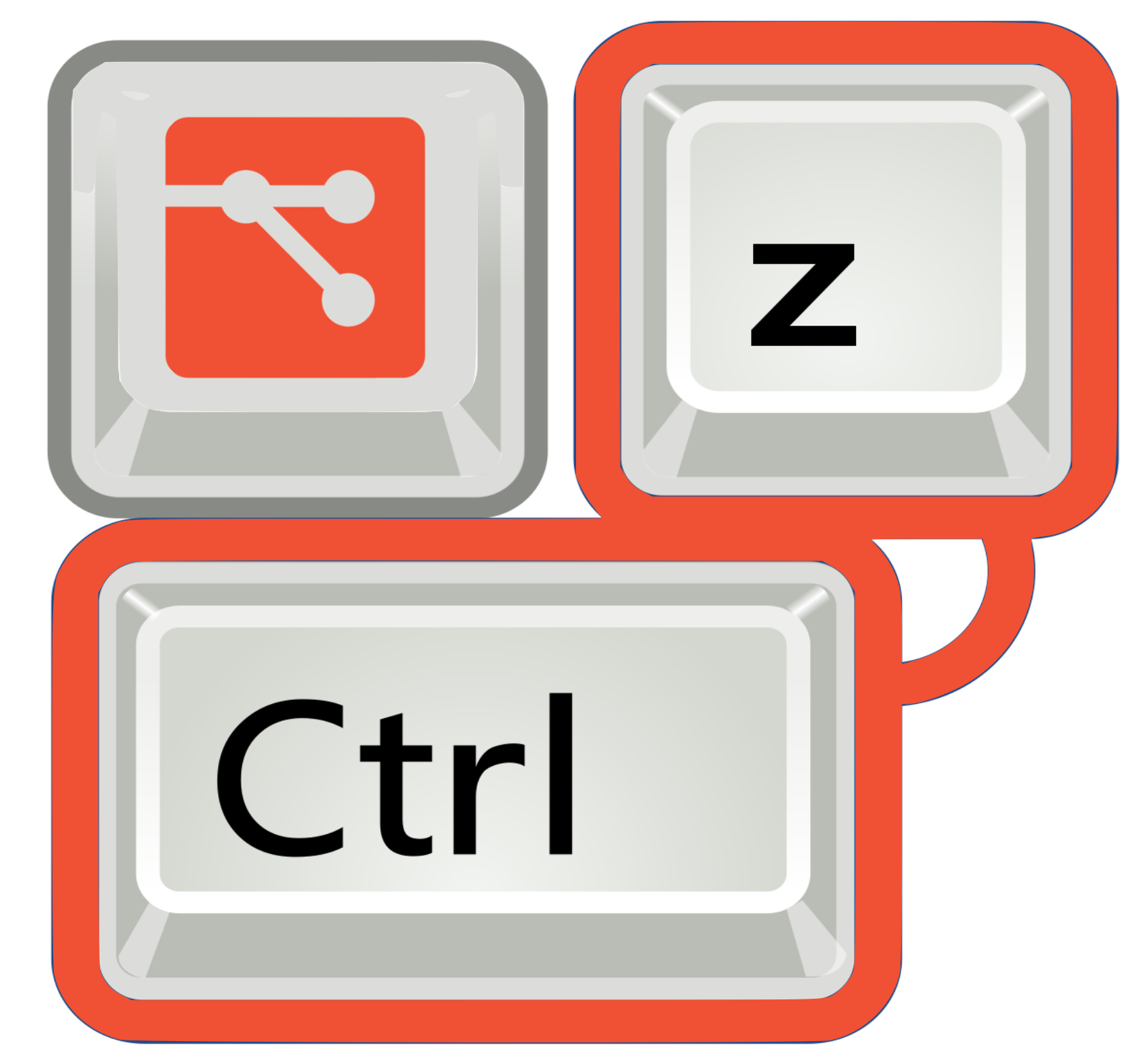# Functional Programming in Python 🐍

📅️ Published: July 3, 2019  • 🕣 1 min readFeatures like `lambda`, `map`, `filter`, `reduce` are generally used to perform functional programming related tasks in Python. Let’s take a quick look on them.

### Lambdas

• Anonymous functions
• No function name,
• They can be passed as function arguments/objects.
• No `return` statment, evaluated exrpession is returned automatically.
• Single line function.

Example :

``````double = lambda x: x*x
print(double(34))

elementList = [34, 56, 78, 90, 0, 12]
doubleList = lambda elementList: [e*e for e in elementList]
print(doubleList(elementList))
``````

### Map

• applies a function to all the items in an input list.
• `map(function_to_apply, list_of_inputs)`.

Example :

``````myList = ["bhupesh", "varshney", "is", "a", "developer"]

capitalize = list(map(lambda x: x.upper(), myList))
print(capitalize)
``````

### Filter

• creates a list of elements for which a function returns `True`.

Example :

``````mylist = [23, 45, 6, 8, 10, 16]
evenList = list(filter(lambda x: x%2 == 0, mylist))
print(evenList)
``````

### Reduce

• accepts a function and a sequence(list/set etc) and returns a single value calculated.
• Initially, the function is called with the first two items from the sequence and the result is returned.
• The function is then called again with the result obtained in step 1 and the next value in the sequence. This process keeps repeating until there are items in the sequence.

Example :

``````from functools import reduce

li = [5, 8, 10, 20, 50, 100]

sum = reduce((lambda x, y: x + y), li)
print(sum)
``````

Tired of fucking up with git everyday?

ugit helps you undo `git` commands with ease. Undo from 15+ git scenarios